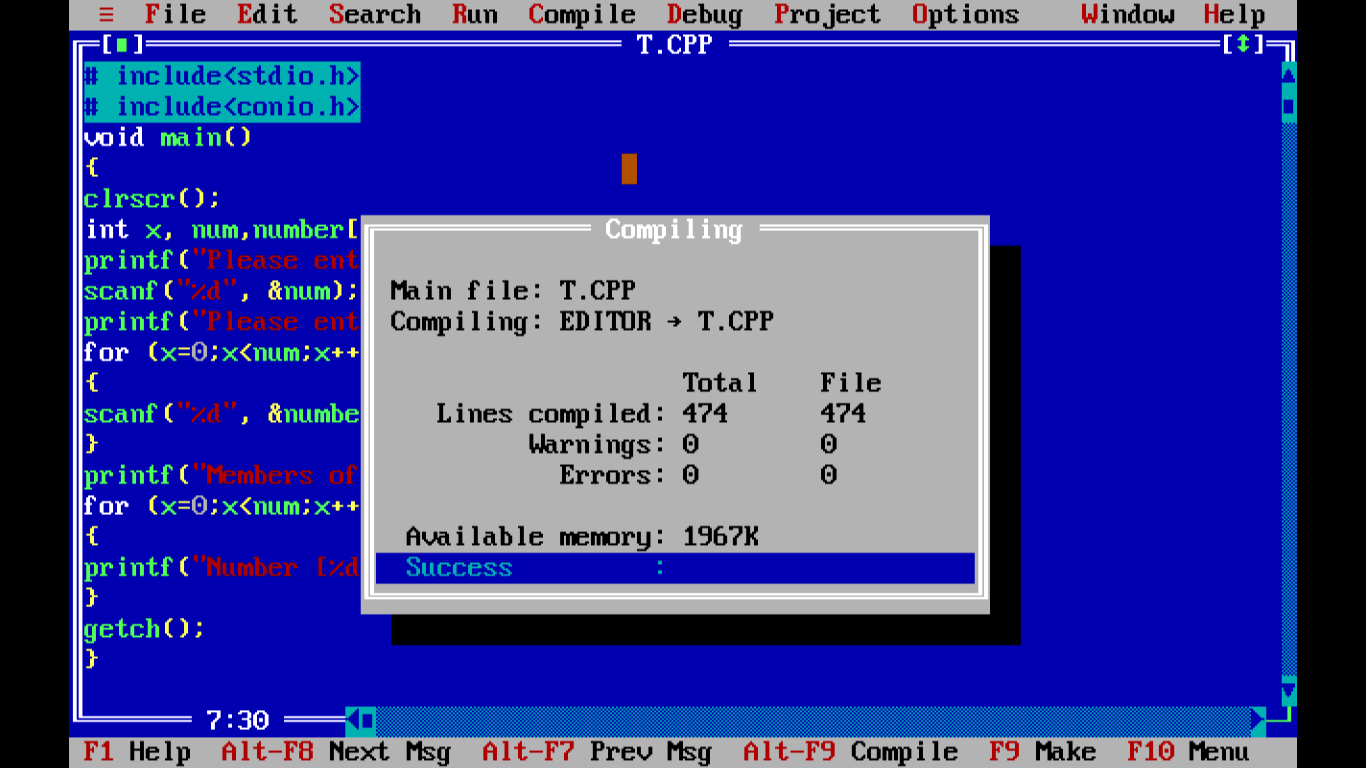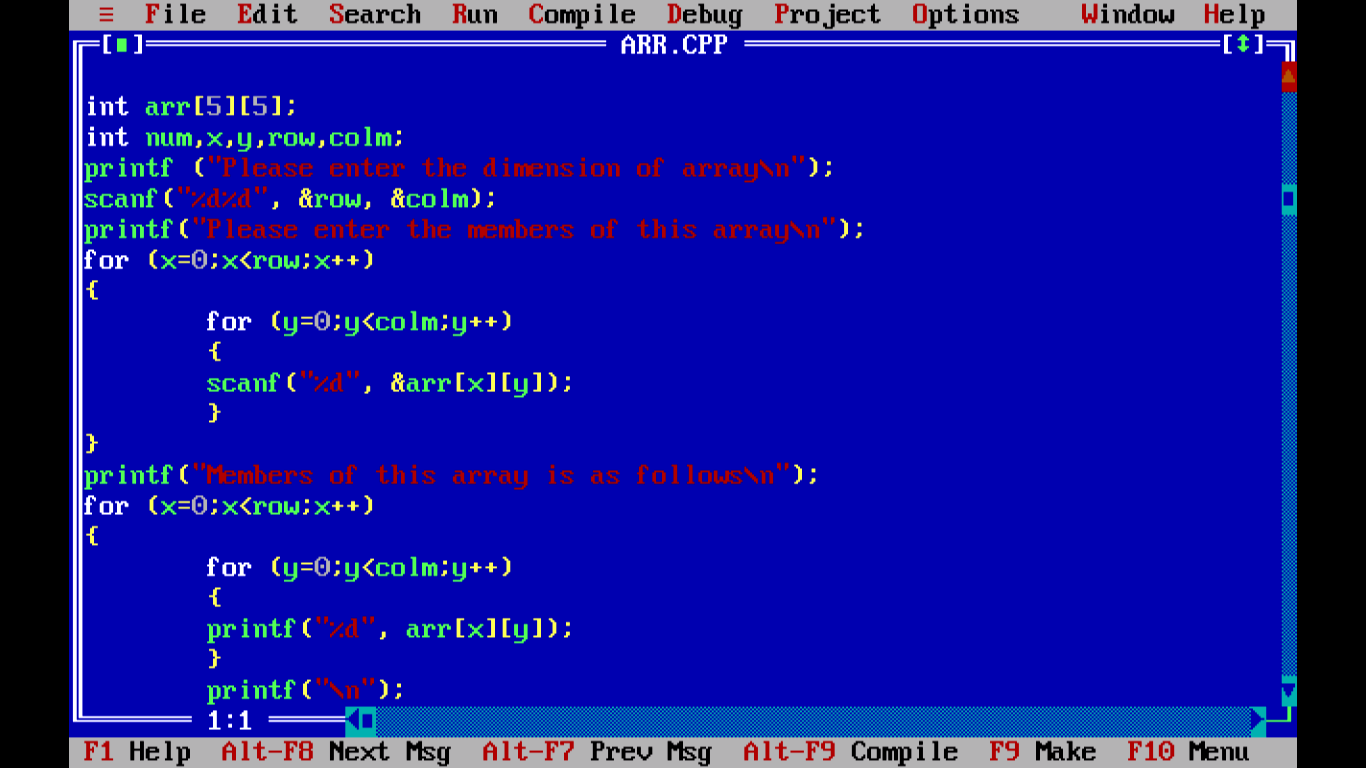# 13 - C Arrays

This chapter will introduce Array in C programming language. We have encountered the term array in the last section also but you must be thinking what actually this array is and how it is so important in programming? SO the answer lies in this chapter. We are aware of variables holding only one data type This programming language facilitates us to design similar data types together and this user defined data type is known as Array. So we can say, array is a collective name assigned to set of similar quantities.

## 13.1 Introduction

We can define array as an ordered group of similar data items. Array stores data in contiguous memory locations in memory. All the elements in an array are of similar type. Now if they are of similar type then how to access each item where each item is of similar data type!! Yes, we can access each item although we have all the items of same type and each item has same name respectively. Array must be declared before using it. We refer them using the position in an array. For example, we have an array of days and we have 7 days in a week so array representing a week can be represented as follows:

/* Program to illustrate single dimensional array */

```# include <stdio.h>
# include <conio.h>

void main()
{
clrscr();
int num, x, number;
printf("Please input the size of array\n");
scanf("%d", &num);
printf("Please enter the elements of array one by one\n");

for (int x=0;x<num;x++)
{
scanf("%d", &number[x]);
}

pruntf("Members of array are as follows\n");
for (x=0;x<num;x++)
{
printf(“Number [%d]=%d\n”, x, number[x]);
}
getch();
}
```Figure  - C Program to illustrate single dimensional arrayFigure - After compiling C Program to illustrate single dimensional arrayFigure - Output of  C Program to illustrate single dimensional array

Let us proceed with multidimensional array.

## 13.1Multidimensional array

Whenever number of subscripts exceeds more than one then this becomes multidimensional array. So such arrays would look as follows:

• Two dimensional array: arr [][]
• Three dimensional array: arr [][][]
• Four dimensional array: arr [][][][]

Format for two-dimensional array :

data type name_of_array [number_of_rows][number_of_columns] ;

Let us consider following example which will demonstrate simplest multidimensional array to you.

/* Program to illustrate multidimensional array */

```# include <stdio.h>
# include <conio.h>

void main()
{
clrscr();
int arr;
int num,x,y,row,colm;

printf("Please enter the dimension of array\n");
scanf("%d%d", &row, &colm);

printf("Please enter the members of this array\n");
for (x=0;x<row;x++)
{
for (y=0;y<colm;y++)
{
scanf("%d", &arr[x][y]);
}
}
printf("Members of this array is as follows\n");
for (x=0;x<row;x++)
for (y=0;y<colm;y++)
{
printf("%d", arr[x][y]);
}

printf("\n");
getch();
}
```Figure – C Program to illustrate multidimensional arrayFigure-  After compiling Program to illustrate multidimensional arrayFigure - Output of  C Program to illustrate multidimensional array

Let us conclude this chapter with this. Next section will introduce pointers. Thank you.